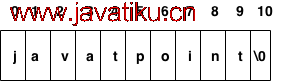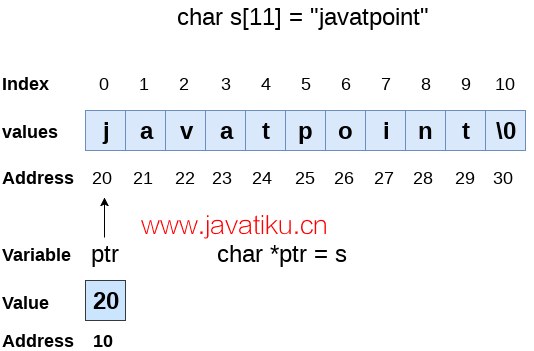# C语言教程-详解C字符串1. 使用字符数组
2. 使用字符串字面值

``char ch = {'j', 'a', 'v', 'a', 't', 'p', 'o', 'i', 'n', 't', '\0'};````char ch[] = {'j', 'a', 'v', 'a', 't', 'p', 'o', 'i', 'n', 't', '\0'};``

``char ch[] = "javatpoint";``

### 字符数组和字符串字面值的区别

• 对于字符数组，我们需要自己在数组末尾添加空字符'0'，而对于字符串字面值，编译器会在末尾自动添加。
• 字符串字面值不能重新分配给另一组字符，而字符数组可以重新分配字符的值。

## C中的字符串示例

``````#include <stdio.h>
#include <string.h>

int main(){
char ch = {'j', 'a', 'v', 'a', 't', 'p', 'o', 'i', 'n', 't', '\0'};
char ch2 = "javatpoint";

printf("Char Array Value is: %s\n", ch);
printf("String Literal Value is: %s\n", ch2);

return 0;
}``````

``````Char Array Value is: javatpoint
String Literal Value is: javatpoint``````

## 遍历字符串

``````#include <stdio.h>

void printString(char *str) {
int i = 0;
while (str[i] != '\0') {
printf("%c", str[i]);
i++;
}
printf("\n");
}

int main() {
char ch[] = "Hello, World!";
printString(ch);
return 0;
}``````

``Hello, World!``

### 使用字符串长度

``````#include<stdio.h>

void main() {
char s = "javatpoint";
int i = 0;
int count = 0;
while (i < 11) {
if (s[i] == 'a' || s[i] == 'e' || s[i] == 'i' || s[i] == 'u' || s[i] == 'o') {
count++;
}
i++;
}
printf("The number of vowels %d", count);
}``````

``The number of vowels 4``

### 使用空字符

``````#include<stdio.h>

void main() {
char s = "javatpoint";
int i = 0;
int count = 0;
while (s[i] != '\0') {
if (s[i] == 'a' || s[i] == 'e' || s[i] == 'i' || s[i] == 'u' || s[i] == 'o') {
count++;
}
i++;
}
printf("The number of vowels %d", count);
}``````

``The number of vowels 4``

## 接受字符串输入

``````#include<stdio.h>

void main() {
char s;
printf("Enter the string?");
scanf("%[^\n]s", s);
printf("You entered %s", s);
}``````

``````Enter the string?javatpoint is the best
You entered javatpoint is the best``````

``````#include<stdio.h>

void main() {
char s;
printf("Enter the string?");
scanf("%[^\n]s", s);
printf("You entered %s", s);
}``````

``````Enter the string?javatpoint is the best
You entered javatpoint is the best``````

### 一些重要注意事项

• 编译器不对字符数组进行边界检查。因此，字符串的长度可能超过字符数组的尺寸，这可能会覆盖一些重要数据。
• 我们可以使用`gets()`而不是`scanf``gets()`是在头文件`string.h`中定义的内置函数。`gets()`只能接收一个字符串。

## 字符串与指针

``````#include<stdio.h>

void main() {
char s = "javatpoint";
char *p = s; // 指针p指向字符串s
printf("%s", p); // 如果打印p，将输出字符串javatpoint
}``````

``javatpoint````````#include<stdio.h>

void main() {
char *p = "hello javatpoint";
printf("String p: %s\n", p);
char *q;
printf("copying the content of p into q...\n");
q = p;
printf("String q: %s\n", q);
}``````

``````String p: hello javatpoint
copying the content of p into q...
String q: hello javatpoint``````

``````#include<stdio.h>

void main() {
char *p = "hello javatpoint";
printf("Before assigning: %s\n", p);
p = "hello";
printf("After assigning: %s\n", p);
}``````

``````Before assigning: hello javatpoint
After assigning: hello``````